# 激光打标中图形和图像处理研究Study on Laser Marking Graphics and Image Processing

DOI: 10.12677/APP.2019.912061, PDF, HTML, XML, 下载: 368  浏览: 771  科研立项经费支持

Abstract: We analyze the image processing problems in the early stage of laser marking and the changes in the marking effect when the setting parameters are adjusted. Based on the requirements of the marking on the image, the image is grayed out, and the grayscale transformation is used to adjust the grayscale range of the original image to present a better visual effect of the marking.

1. 前言

2. 数字图像处理

$f\left(x,y,z\right)=\left\{{f}_{red}\left(x,y,z\right),{f}_{green}\left(x,y,z\right),{f}_{blue}\left(x,y,z\right)\right\}$ (1)

$f\left(x,y,z\right)=\left\{{f}_{red}\left(x,y\right),{f}_{green}\left(x,y\right),{f}_{blue}\left(x,y\right)\right\}$ (2)

$\left[\begin{array}{cccc}f\left(0,0\right)& f\left(0,1\right)& \cdots & f\left(0,N-1\right)\\ f\left(1,0\right)& f\left(1,1\right)& \cdots & f\left(1,N-1\right)\\ ⋮& ⋮& \ddots & ⋮\\ f\left(M-1,0\right)& f\left(M-1,1\right)& \cdots & f\left(M-1,N-1\right)\end{array}\right]$ (3)

3. 位图和矢量图转换

$f\left(i,j\right)=\left\{\begin{array}{l}0\text{}\text{\hspace{0.17em}}\text{}f\left(i,j\right) (4)

4. 激光打标效果的影响因素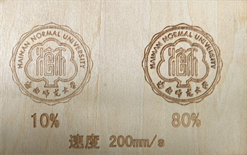(a)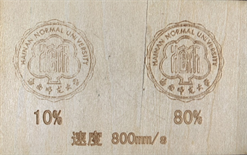(b)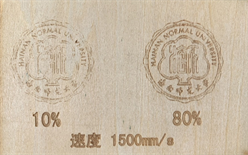(c)

Figure 1. (a) The marking speed of 200 mm/s; (b) The marking speed of 800 mm/s; (c) The marking speed of 1500 mm/s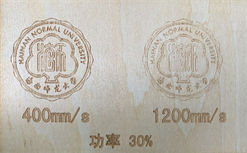(a)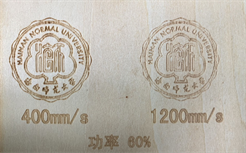(b)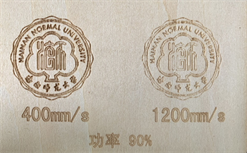(c)

Figure 2. (a) The laser output power of 30%; (b) The laser output power of 60%; (c) The laser output power of 90%

5. 总结

  王力何, 马殿光. 激光打标机的发展趋势及其控制系统设计[J]. 机电信息, 2015, 429(3): 102-104.  姚蔡翔. 激光加工的原理、应用现状与展望[J]. 山东工业技术, 2013(15): 12-14.  宋琳琳. 计算机图形与图形图像处理技术的相互结合分析[J]. 信息通信, 2014(8): 125.  孙文. 计算机技术在图形图像处理中的应用及关键技术[J]. 电子技术与软件工程, 2018(10): 56.  陈冬梅, 王宏宇. 计算机技术在图形图像处理中的应用及关键技术研究[J]. 电子测试, 2017(7): 120-121.  宁国勤, 朱中煜, 朱绍文, 肖毅. 激光打标中图形、图像的处理[J]. 激光技术, 2002, 26(4): 295-296.  李仪, 潘佑华, 陈平平. 激光打标机聚焦性能的优化设计[J]. 激光与光电子学进展, 2011(48).  王建平, 李正佳, 范晓红. 激光打标系统及工艺参数的分析[J]. 光学与光电技术, 2005, 3(3): 33-35.